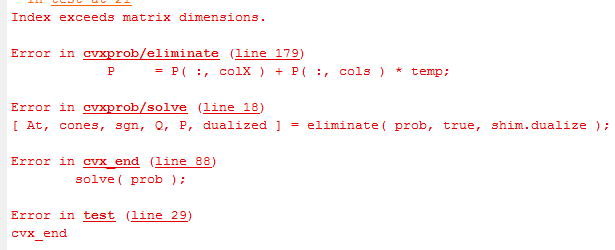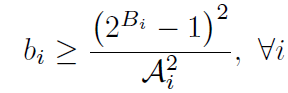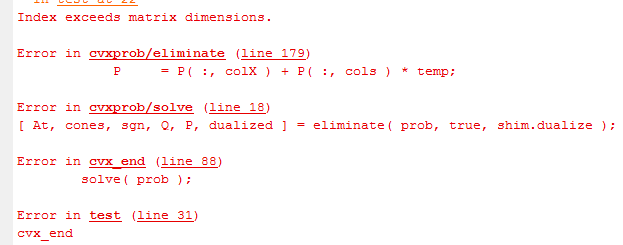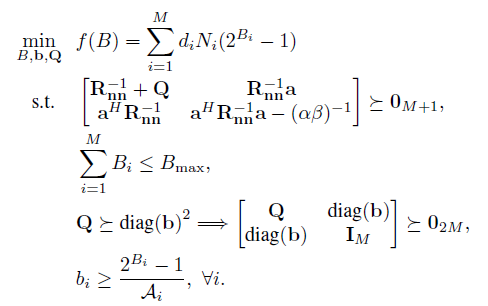# CVX_SDP Index exceeds matrix dimensions problem

Hi, everyone, I have an optimization problem,which is obtained by convex relaxation.
Then, I write code in matlab based on CVX as follows:

clc;clear all
M = 50;
R = rand(M,M);
R = RR’;
c0 = rand(M,1);
i = sqrt(-1)
as = rand(M,1) + i
rand(M,1);
lambda = 10;
Alength = rand(M,1);
R = R + i*R;
invRnn = inv®;
Bmax = 1;

cvx_begin sdp
variable B(M,1)
variable b(M,1)
variable t(M,1)
minimize ( sum(c0.(2.^B)) )
subject to
[invRnn + diag(b) invRnn
as; as’*invRnn as’invRnnas-lambda] >= zeros(M+1, M+1)
sum(B) <= Bmax
for i = 1:M
% b(i,1) >= (2^B(i)-1)^2/Alength(i)^2;
[1, t(i); t(i), b(i,1)*Alength(i,1)^2] >= 0
t(i) >= 2^B(i,1)-1
end
% b >= ((2.^B-1)./Alength).^2
cvx_end
stem(B)

But I cannot get the solution, matlab always give me an error message asSo who knows how to correct the error? Thanks in advance.

How to write this constraint in CVXActually, b, B, A are vectors of dimension M.

I try to write like this:
for i = 1:M
% b(i,1) >= (2^B(i)-1)^2/Alength(i)^2;
[1, t(i); t(i), b(i,1)*Alength(i,1)^2] >= 0
t(i) >= 2^B(i,1)-1
end
But CVX gives me the error message asWho can tell me why? Thanks in advance.

You probably triggered a bug in CVX (I encountered something similar once). Can you shorten your program by setting M as small as possible, replacing calls to `rand` by explicit matrices, remove constraints… and still trigger the bug?

If you provide a shorter test case that exhibits the problem, it’s easier to identify the bug.

Or, if you just care about results, move things around, reformulate, change the type of constraints, until CVX works correctly.

I’m having a lot of trouble reproducing this issue, honestly. But one thing that does seem to be in common across all the instances of this is that it involves a combination of semidefinite variables and log/exp/power functions. If anyone can offer a reliable, fully self-contained way to reproduce this problem, I can keep looking for a solution.

Hello, everyone. I have an optimization problem as followsThen, I wrote the codes in MATLAB, as follows

Why matlab always shows the error like thisI don’t understand the reason, because I have checked that there is not problem with matrix dimension.
So who can give me advice? thanks very much.

Hope someone can give advice on solving this problem. Thanks a lot. Awaiting…

Hi, everybody,

I’m trying to solve a problem with CVX in SDP mode such as follows:

cvx_begin sdp
variables Ps(N) Psi(N);
variable W11(N,N) hermitian toeplitz;
variable W22(N,N) hermitian toeplitz;
minimize -(sum(log(1+Psi.*r1)))
subject to
[W11, diag(Psi);diag(Psi), W22]>=0;
cvx_end

where N is a constant, r1 is constant vector.

When N<=16, it can work. However, when N beomes larger (e.g. N=32), it will fail. The errors are listed as follows:

??? Index exceeds matrix dimensions.

error in ==> cvxprob/eliminate (line 170)
P = P( :, colX ) + P( :, cols ) * temp;

eroor in ==> cvxprob/solve (line 14)
[ At, cones, sgn, Q, P, dualized ] = eliminate( prob, true );

error in ==> cvx_end (line 77)
solve( prob );

error in ==> test0 (line 154)
cvx_end

I cann’t find out what’s wrong with it. Can anybody help me? Thanks a lot.

Hi, have you known the reason behind your problems? I have a similar problem, see CVX_SDP Index exceeds matrix dimensions problem. Maybe you can help me.

I’m going to merge these common issues into a single thread.

Just to reiterate what I said above: I’m having trouble reproducing this issue. I need a complete, self-contained example—no missing data that I have to guess about! And preferably, one that is as small as possible. If someone can offer that up, I’d be grateful, as would (I suspect) those who are suffering from this bug! Thank you!

Hi, have you solved your problem?

Hi everyone!
I encountered the same problem but I have some information that might help you guys resolve this issue and fix the bug.

So, I tried to minimize the following function:under the constraint Ax=b. I tried to do this with the following MATLAB code:

clear all;
close all;
clc;

n = input(‘n=’);
p = input(‘p=’);

A = rand(p,n);
x0 = rand(n,1);
b = A*x0;

cvx_begin
variable x(n)
minimize (-sum(log(x)))
subject to
A*x==b;
cvx_end

I tried to do this for various combinations of n and p. I noticed that the minimization was completed successfully every time p was smaller than 2n. When p was bigger than 2n I also got the same error message as you guys, that is:

Index exceeds matrix dimensions.

Error in cvxprob/eliminate (line 179)
P = P( :, colX ) + P( :, cols ) * temp;

Error in cvxprob/solve (line 18)
[ At, cones, sgn, Q, P, dualized ] = eliminate( prob, true, shim.dualize );

Error in cvx_end (line 88)
solve( prob );

Error in ConvexOptimization3 (line 13)
cvx_end

I use MATLAB R2015a in Ubuntu 16.04 LTS. You are welcome to try this code by yourselves and see if this information about the p and n parameters helps you find the bug.

Hi,
have you known the reason behind this issue? I have a similar problem.
In particular, I have an optimization problem with a constraint which includes the log determinant of a matrix A, A is a linear combination of the PSD matrices and optimization scalar variables, as -log_det (A)<t, (t is a constant). Matlab always shows the same error as follows. I don’t understand the reason, because I have checked that there is not problem with matrix dimension. And for example, as a test, when I change log_det (A) to trace (A), there is not any error.

Error using *
Incorrect dimensions for matrix multiplication. Check that the number of columns in the first matrix matches the number of rows in the
second matrix. To perform elementwise multiplication, use ‘.*’.

Error in cvxprob/eliminate (line 137)
P = P * cvx_invert_structure( xR );

Error in cvxprob/solve (line 18)
[ At, cones, sgn, Q, P, dualized ] = eliminate( prob, true, shim.dualize );

Error in cvx_end (line 88)
solve( prob );

@Zahra1 Can you provide a complete reproducible problem? Are you using CVX 2.2? Does the error occur if you use a different solver?

It appears that this bug, whatever it is, might not have been fixed.

Thank you very much. I will short the code and then send it.
Yes, I am using CVX 2.2. Yes it occurs with different slovers.
One thing is that, my problem has different constriants. I noticed that the errors occures when more than one constraint includes the term log_det (A).

You should submit reproducible problems as bug report per instructions at http://cvxr.com/cvx/doc/support.html . No guarantee what the follow through will be, though (I am not involved in dealing with bug reports).